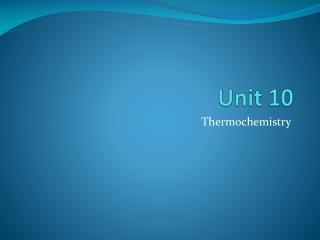DownloadDownload PresentationUnit 10

# Unit 10

Télécharger la présentation## Unit 10

- - - - - - - - - - - - - - - - - - - - - - - - - - - E N D - - - - - - - - - - - - - - - - - - - - - - - - - - -
##### Presentation Transcript

1. Unit 10 Thermochemistry

2. Energy • Energy: ability to do work or produce heat. • Kinetic energy: energy of motion • Potential energy: due to composition or position of an object. • Chemical systems contain both kinetic and potential energy. • Law of Conservation of energy: states that in an y chemical reaction or physical process, energy can be converted from one form to another, but is neither created or destroyed. • Heat (q): energy that flows from a warmer object to a cooler object. • If object loses heat, temperature decreases and if it gains heat, temperature increases.

3. Measuring heat • calorie (cal): the amount of heat required to raise the temperature of 1g of water by 1C. • Nutritional Calorie (Cal): 1Calorie= 1000 calories= 1kcal • SI unit for measuring heat: joule ( J) 1 cal = 4.184 J

4. Specific heat (c) • Amount of heat required to raise the temperature of one gram of that substance by 1C. • Units: J/g C or cal/g C

5. Calculating heat released and absorbed. • q = mcT • q: heat (J or cal) • m: mass (g) • c: specific heat (J/g C) or (cal/g C) •  T: change in temperature • T = Tfinal - Tinitial

6. Example 1 • In the construction of bridges and sky scrapers, gaps must be left between adjoining steel beams to allow for expansion and contraction of the metal due to heating and cooling. The temperature of a sample of iron with a mass of 10.0g changed from 55.0C to 85.0C with the release of 114 J of heat. What is the specific heat of iron? • q=mcT q= 114J m= 10.0g T=(85-55)= 30C 114J= 10.0 g x c x 30C c = 0.38 J/g  C

7. Example 2. • If the temperature of 34.4g of ethanol increases from 25.0 C to 78.8 C, how much heat is being absorbed by the ethanol? C ethanol= 2.44 J/g C • Answer: 4516 J

8. Example 3 • How many joules of heat are lost by 3580 kg of granite as it cools from 41.2 C to -12.9C? cgranite = 0.803 J/gC 1 kg= 1000g

9. Heat in chemical reactions and processes • Measuring heat: • Calorimeters : are used to measure heat or calculate specific heat

10. Measuring heat • The heat released by the system is equal to the heat absorbed by its surroundings. • System: part of the universe on which you focus your attention • Surroundings: includes everything else in the universe. qsystem= -qsurrounding q= mcT

11. Ex. 1 A small pebble is heated and placed in a foam cup calorimeter containing 25.0g of water at 25.0C. The water reaches a maximum temperature of 26.4C. How many joules of heat were released by the pebble? (cwater=4.184 J/g C) qwater= mcT = 25.0g 4.184 J (26.4 C- 25.0C) g  C = 146 J qsystem= -qsurrounding qwater= - qpebble qpebble= -qwater= - 146J

12. Ex. 2 • An alloy of unknown composition is heated to 137 °C and placed into 100.0 g of water at 25.0 °C. If the final temperature of the water and alloy was 36.4 °C, and the alloy weighed 2.71 g, what is the specific heat of the alloy? The specific heat of water is 4.184 J/gºC.

13. Learning Check 1 • Given that the specific heat of gold is 0.129 J/gºC, calculate the final temperature for the system if a 200.0 g block of gold at 100.0 ºC is placed in a coffee-cup calorimeter containing 50.0 g of water at an initial temperature of 25.0 ºC. The specific heat of water is 4.184 J/gºC. (Answer: 33.2C)

14. Thermochemical equations • Most chemical reactions and physical changes occur at constant pressure. • The heat content of a system at constant pressure is called enthalpy (H) of the system. • The heat absorbed or released by a reaction at constant pressure is the same as the change in enthalpy or H. • For us: q= H

15. Thermochemical equation: balanced chemical equation that includes physical states of reactants and products and the energy change (H) • Ex 4Fe(s) + 3 O2 (g)  2Fe2O3 (s) H= -1625 kJ • H is negative for EXOTHERMIC reactions • H is positive for ENDOTHERMIC reactions

16. ENERGY AND CHANGES OF STATE • Enthalpy of fusion (Hfus): energy required to melt 1 gram (or 1 mole) of a substance at its melting point. • For water: Hfus=334J/g or 6.01kJ/mol • Enthalpy of solidification Hsolid : Hfus = - Hsolid • Enthalpy of vaporization (Hvap): energy required to vaporize 1 gram (or 1 mole) of a substance at its boiling point. • For water: Hvap=2260J/g or 40.7kJ/mol • Enthalpy of condensation Hcond : Hvap = - Hcond • During a change of state, there is no change in temperature.

17. Ex.1 How much heat (in kJ) is absorbed when 24.8g H2O(l) at 100C is converted to steam at 100 C? • For water: Hvap= 40.7kJ/mol • mwater= 24.8 g • Calculate molar mass water: 18g/mol 24.8g 1 mol 40.7 kJ 18g 1 mol = 56.1 kJ

18. How much heat is required to melt 5.67g of iron (II) oxide, FeO, if its Hfus= 32.2 kJ/mol? • Hfus= 32.2 kJ/mol? • m= 5.67 g FeO • Molar mass= 71.8g/mol 5.67g FeO 1 mol 32.2 kJ 71.8 g 1 mol = 2.54 kJ